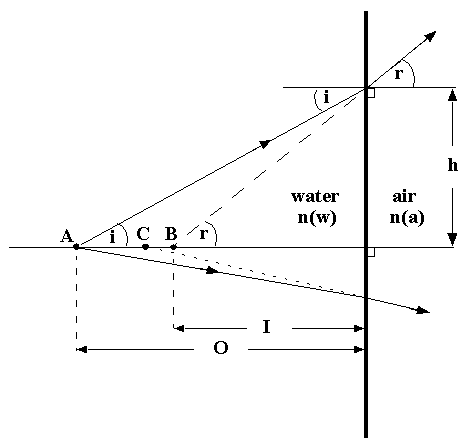# Planar Interfaces and Apparent Positions

In-water objects viewed through a planar air/water interface, such as a mask or flat port of a camera housing, appear closer than they really are. This is due to refraction at the interface, and because the index of refraction of water is greater than that of air. Consider a point object at A, which is a perpendicular distance O from the interface, and a light ray from A incident on the interface at angle 'i', intersecting it at a height 'h' . If the indices of refraction of water and air are n(w) and n(a), respectively, the angle of refraction 'r' is given by Snell's law:

`sin(i)/sin(r) = n(a)/n(w)             (Equation 1)`

`I = O*tan(i)/tan(r)                    (Equation 2)``I = O*[n(a)/n(w)]*cos(r)/cos(i)        (Equation 3)`

`I = O*n(a)/n(w)                       (Equation 4)`